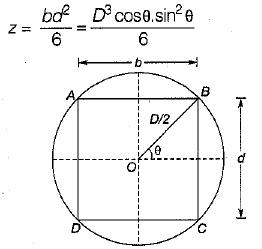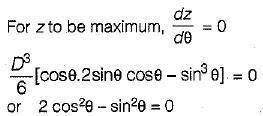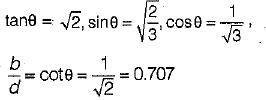Courses

# Properties Of Metals, Simple Stress Strain And Elastic Constants - 1

## 10 Questions MCQ Test Mock Test Series for Civil Engineering (CE) GATE 2020 | Properties Of Metals, Simple Stress Strain And Elastic Constants - 1

Description
This mock test of Properties Of Metals, Simple Stress Strain And Elastic Constants - 1 for Civil Engineering (CE) helps you for every Civil Engineering (CE) entrance exam. This contains 10 Multiple Choice Questions for Civil Engineering (CE) Properties Of Metals, Simple Stress Strain And Elastic Constants - 1 (mcq) to study with solutions a complete question bank. The solved questions answers in this Properties Of Metals, Simple Stress Strain And Elastic Constants - 1 quiz give you a good mix of easy questions and tough questions. Civil Engineering (CE) students definitely take this Properties Of Metals, Simple Stress Strain And Elastic Constants - 1 exercise for a better result in the exam. You can find other Properties Of Metals, Simple Stress Strain And Elastic Constants - 1 extra questions, long questions & short questions for Civil Engineering (CE) on EduRev as well by searching above.
QUESTION: 1

### A material has identical properties in all directions, it is said to be

Solution:

Isotropic- Identical properties in all directions
Orthotropic- Different properties in all three directions
Homogeneous- A material of uniform composition

QUESTION: 2

### The term nominal stress in stress-strain curve for mild steel implies

Solution: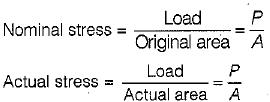Actual area at instant of loading does not remain constant and decreases with increases in elongation actual stress is also called true stress.

QUESTION: 3

### For metallic minerals creep becomes an important consideration at

Solution:

The temperature at which the creep becomes an important consideration is called HOMOLOGOUS TEMPERATURE and this temperature is nearly half of the melting point temperature.

QUESTION: 4

Clapeyron's theorem is associated with the analysis of

Solution:

Clapeyorn’s Theorem of Three Moments express the relation b/w bending moments at three successive supports of a continuous beam, subjected to a loading on two adjacent span with or without settlement of supports.

QUESTION: 5

A rubber band is elongated to double its initial length, its true strain is

Solution: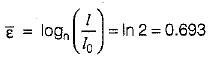QUESTION: 6

A prismatic beam has uniform

Solution: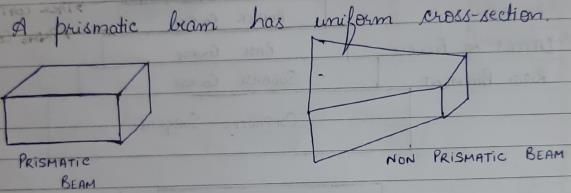QUESTION: 7

In the case of pure bending, the beam will bend into an arc of a

Solution:

In case of pure moment, bending moment at any section will be same. Thus, using double integration method to find deflection we get the equation of parabola.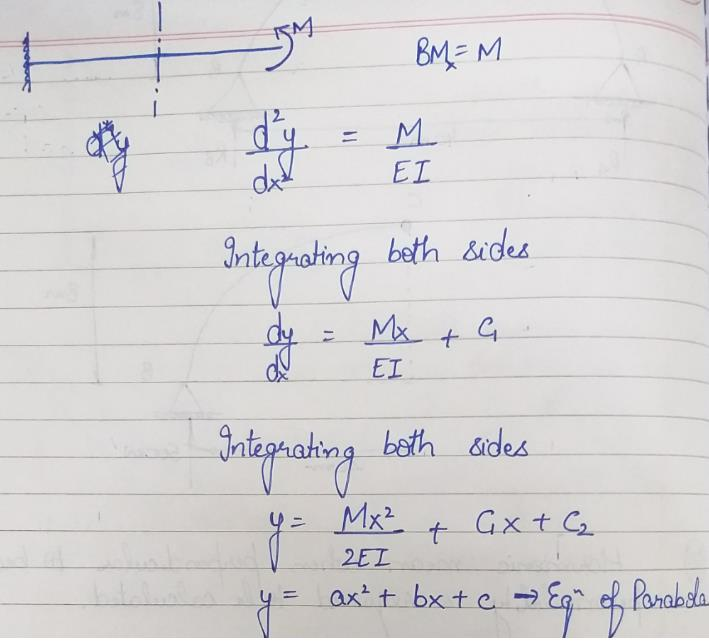QUESTION: 8

If the modulus of elasticity is zero, the material is said to be

Solution:

E =0  Slope of Stress- Strain Curve is straight horizontal line. It means at constant stress, strain keeps increasing which is the characteristic property of perfectly plastic material.

QUESTION: 9

The compressibility of a material is proportional to

Solution: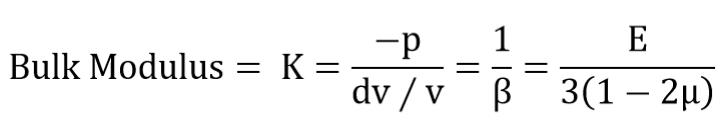Bulk Modulus= K=(-p)/(ⅆv∕v)=1/β=E/3(1-2μ)

β=compressibility is proportional to reciprocal of E.

QUESTION: 10

If a beam with the rectangular cross-section is obtained by cutting from circular log of timber, then for the beam to have strongest section in bending, the ratio of breadth to depth should be

Solution:

AB = b = D cosθ, BC = d = D sinθ